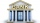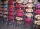Pamela

Lara Pamela spends her salary of 3000 for food, clothing, recreation and savings, which are in the ratio of 48:20:15:37, respectively. How much does he spend for savings?

Result

x =  925

Solution:Leave us a comment of this math problem and its solution (i.e. if it is still somewhat unclear...):Be the first to comment!To solve this verbal math problem are needed these knowledge from mathematics:

Need help calculate sum, simplify or multiply fractions? Try our fraction calculator.

Next similar math problems:

1. SpendingPeter spends 1/5 of his earnings on his rent and he saves 2/7. What fraction of his earnings is left?Cris had 15000 . He spent 1/3 of his money on a table and the remaining on 5 similar chairs that cost the same. How much did one chair cost?
3. HusseinHussein owns 450000. He spent at the bookstore 2 over 9 to buy some books and tales. He paid 3 over 5 of his money to buy his math book. Then he paid his money to buy stories diagram ; a. Calculate the remaining amount of money with Hussein? b. Hussein l
4. Fraction to decimalWrite the fraction 3/22 as a decimal.
5. AndreAndre, Thomas, and Ivan split 88 postage stamps in a 2:5:4 ratio. How much did Thomas get?
6. Write 2Write 791 thousandths as fraction in expanded form.
7. Ratio 11Simplify this ratio 10 : 1/4
8. Cupcakes 2Susi has 25 cupcakes. She gives 4/5. How much does she have left?
9. In fractionsAn ant climbs 2/5 of the pole on the first hour and climbs 1/4 of the pole on the next hour. What part of the pole does the ant climb in two hours?
10. TeacherTeacher Rem bought 360 pieces of cupcakes for the outreach program of their school. 5/9 of the cupcakes were chocolate flavor and 1/4 wete pandan flavor and the rest were a vanilla flavor. How much more pandan flavor cupcakes than vanilla flavor?
11. Lengths of the poolMiguel swam 6 lengths of the pool. Mat swam 3 times as far as Miguel. Lionel swam 1/3 as far as Miguel. How many lengths did mat swim?
12. PointsGryffindor won 437 points. How many points obtained by each of the faculties if they were split at a ratio of 5: 7: 3: 4?
13. RunnersFor three runners (on the first to third place) is prepared 30 chocolate that they be distributed in the ratio of 3 : 2 : 1 How much chocolate will get everyone?
14. BuildingAt the building, we divided 240 boards into two piles in a 5: 3 ratio. How many were fewer boards in the lower pile?
15. Fraction and a decimalWrite as a fraction and a decimal. One and two plus three and five hundredths
16. PercentagesWhat is 1/3 of \$821.43?
17. Divide moneyDivide 1200 USD at a ratio of 1:2:3:4:5:6:9:10